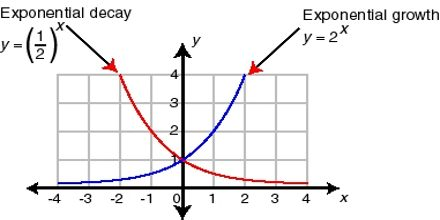Mathematic

# Exponential Growth and DecayExponential Growth and Decay are functions which have been widely used to model the behavior of a variety of topics. Primary objective of this lecture is to present on Exponential Growth and Decay. At the Algebra level, there are two functions that can be easily used to illustrate the concepts of growth or decay in applied situations. When a quantity grows by a fixed percent at regular intervals, the pattern can be represented by the functions, Growth: y = a (1+r)² and Decay: y = a (1-r)².